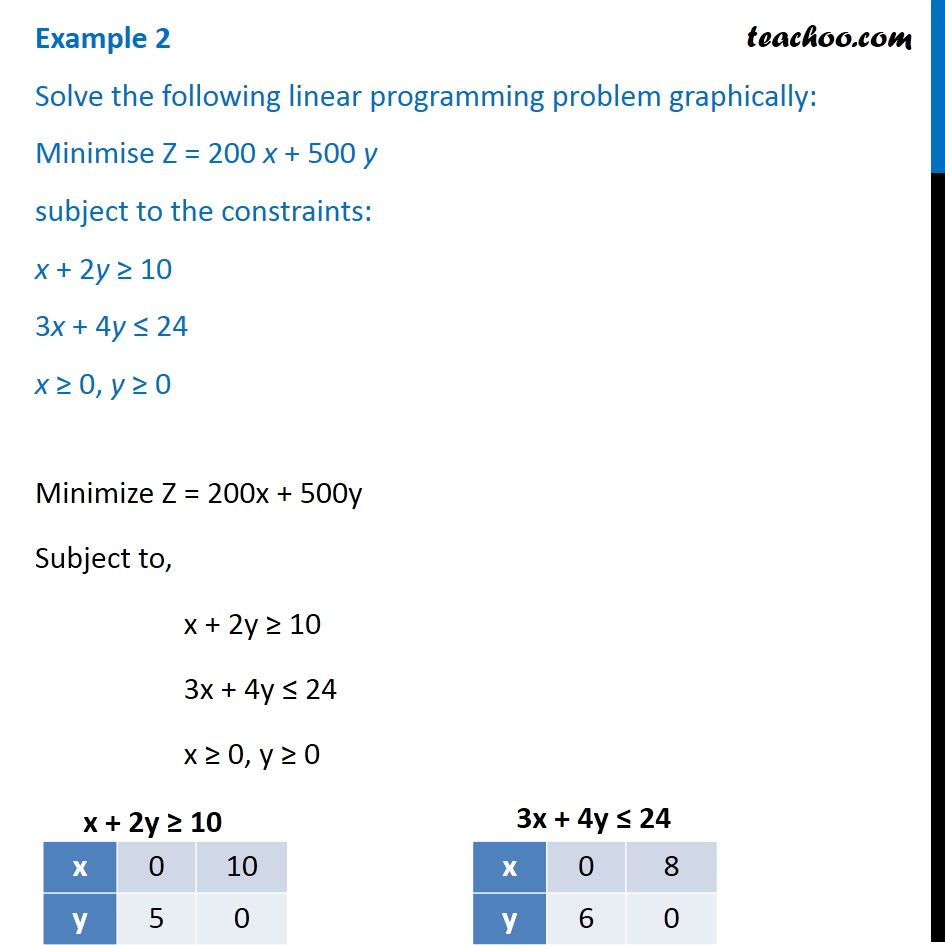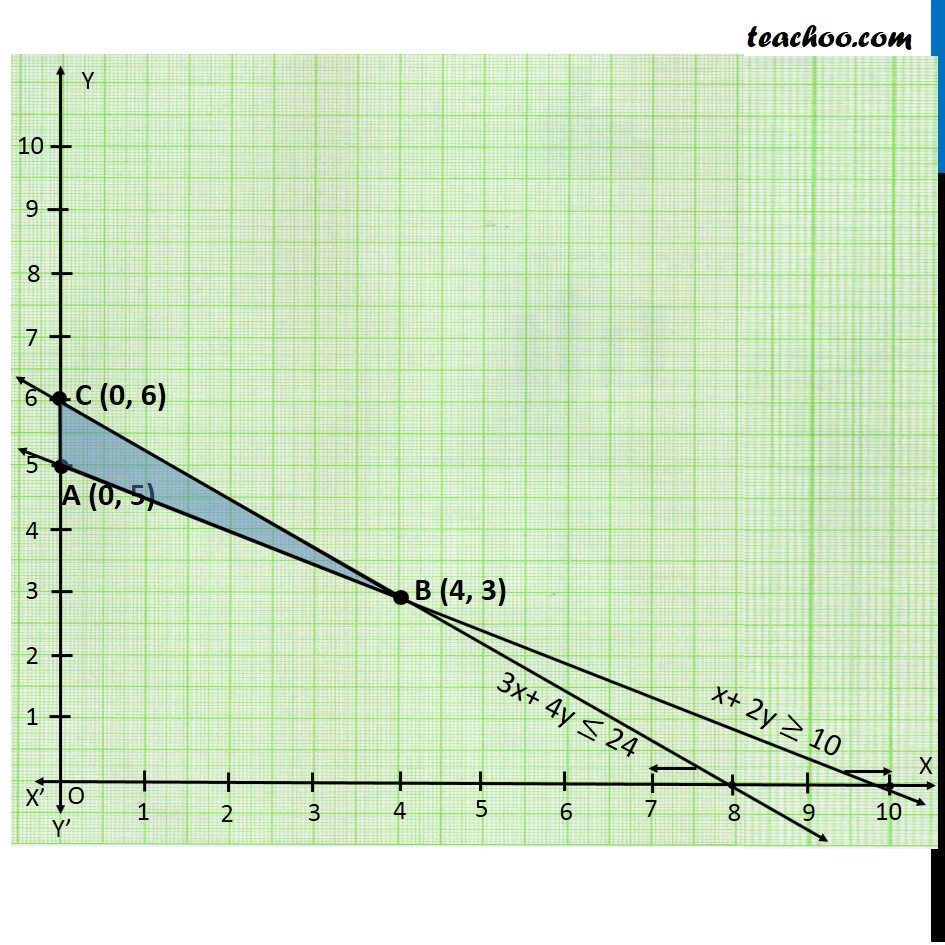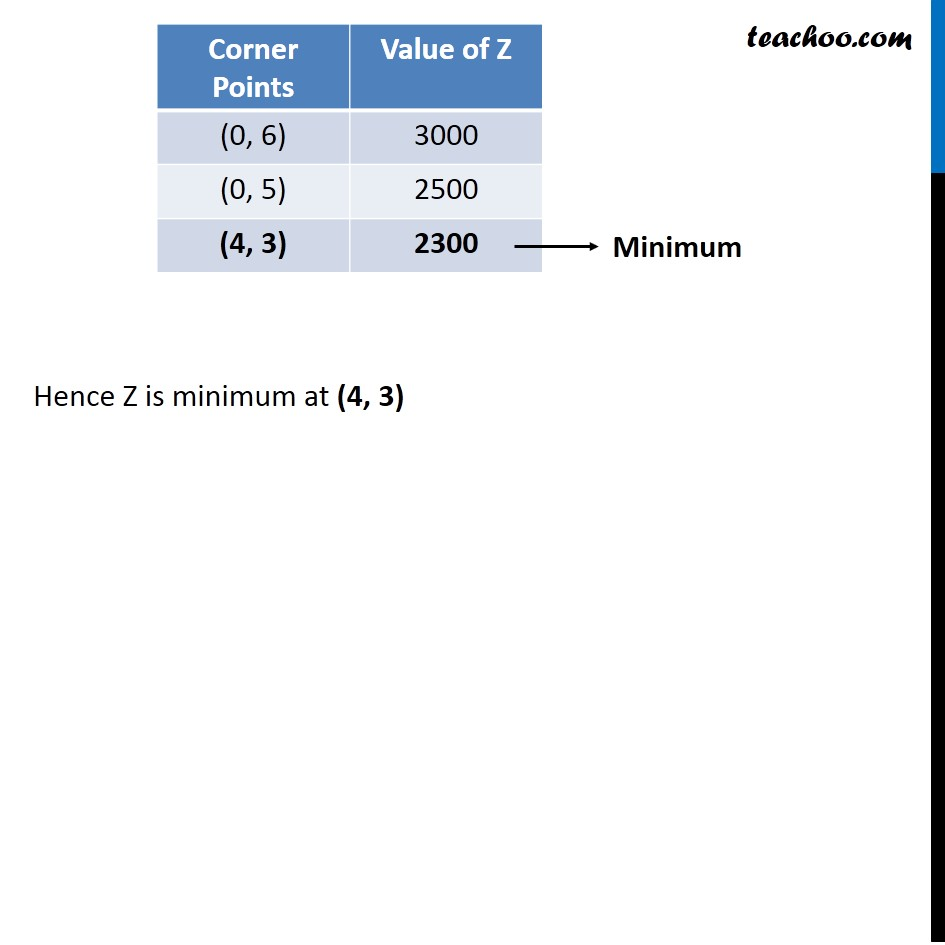Linear equations given - Bounded

Chapter 12 Class 12 Linear Programming
Concept wiseIntroducing your new favourite teacher - Teachoo Black, at only ₹83 per month

### Transcript

Example 2 Solve the following linear programming problem graphically: Minimise Z = 200 x + 500 y subject to the constraints: x + 2y ≥ 10 3x + 4y ≤ 24 x ≥ 0, y ≥ 0 Minimize Z = 200x + 500y Subject to, x + 2y ≥ 10 3x + 4y ≤ 24 x ≥ 0, y ≥ 0 Hence Z is minimum at (4, 3)